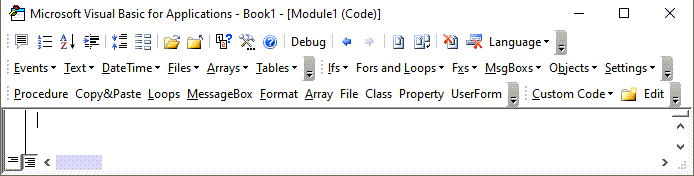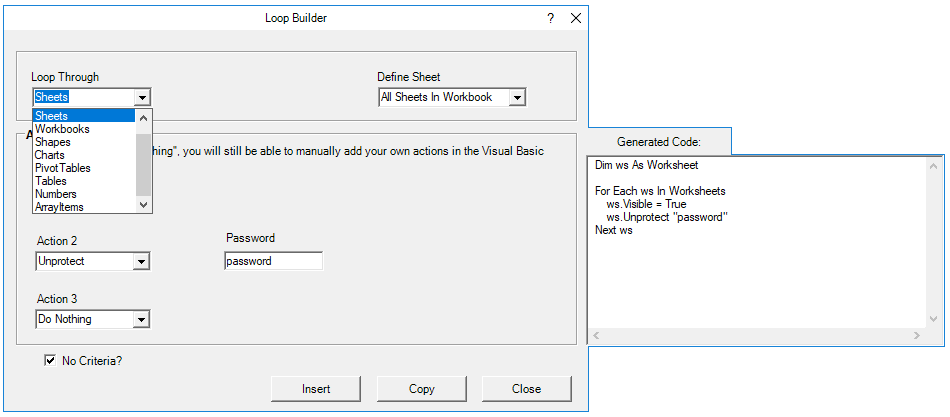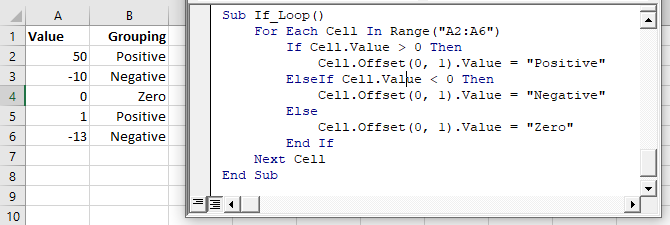# VBA For Each Examples (Quick Reference)

## For Each Loop

The For Each Loop allows you to loop through each object in a collection:

• All cells in a range
• All worksheets in a workbook
• All open workbooks
• All shapes in a worksheet
• All items in an array
• and more!

## For Each: Basic Examples

These examples will demonstrate how to set up For Each loops to loop through different types of objects.

### Loop Through Cells

This procedure will loop through each cell in range A1:A10, setting the cell to it’s right equal to itself.

``````Sub ForEachCell()
Dim Cell As Range

For Each Cell In Sheets("Sheet1").Range("A1:A10")
Cell.Offset(0, 1).value = Cell.value
Next Cell

End Sub``````

### Loop Through Sheets

This procedure will loop through each sheet in a Workbook, unhiding each sheet.

``````Sub ForEachSheets()
Dim ws As Worksheet

For Each ws In Sheets
ws.Visible = True
Next ws

End Sub``````

### Loop Through Workbooks

This procedure will loop through each Workbook, closing each one.

``````Sub ForEachWorkbooks()
Dim wb As Workbook

For Each wb In Workbooks
wb.Close
Next wb

End Sub``````

### Loop Through Shapes

This procedure will loop through each shape in Sheet1, deleting each one.

``````Sub ForEachShape()
Dim Shp As Shape

For Each Shp In Sheets("Sheet1").Shapes
Shp.Delete
Next Shp

End Sub``````

Stop searching for VBA code online. Learn more about AutoMacro - A VBA Code Builder that allows beginners to code procedures from scratch with minimal coding knowledge and with many time-saving features for all users!### Loop Through Charts

This procedure will loop through each Chart in Sheet1, deleting each one.

``````Sub ForEachCharts()
Dim cht As ChartObject

For Each cht In Sheets("Sheet1").ChartObjects
cht.Delete
Next cht

End Sub``````

### Loop Through PivotTables

This procedure will loop through each PivotTable in Sheet1, clearing each one

``````Sub ForEachPivotTables()
Dim pvt As PivotTable

For Each pvt In Sheets("Sheet1").PivotTables
pvt.ClearTable
Next pvt

End Sub``````

### Loop Through Tables

This procedure will loop through each Table in Sheet1, deleting each one.

``````Sub ForEachTables()
Dim tbl As ListObject

For Each tbl In Sheets("Sheet1").ListObjects
tbl.Delete
Next tbl

End Sub``````

VBA Programming | Code Generator does work for you!

### Loop Through Items in Array

This procedure will loop through each item in an Array, display each value in a msgbox,

``````Sub ForEachItemInArray()
Dim arrValue As Variant
Dim Item As Variant
arrValue = Array("Item 1", "Item 2", "Item 3")

For Each Item In arrValue
MsgBox Item
Next Item

End Sub``````

### Loop Through Numbers

This procedure will loop through each number in an Array, display each value in a msgbox,

``````Sub ForEachNumberInNumbers()
Dim arrNumber(1 To 3) As Integer
Dim num As Variant

arrNumber(1) = 10
arrNumber(2) = 20
arrNumber(3) = 30

For Each num In arrNumber
Msgbox num
Next num

End Sub``````

## For Each Loop BuilderThe Loop Builder makes it very easy to generate code to loop through objects.  AutoMacro also contains many other Code Generators, an extensive Code Library, and powerful Coding Tools.

## For Each – If

You can also use If Statements within Loops to test if objects meet certain criteria, only performing actions on those objects that meet the criteria.  Here is an example of looping through each cell in a range:

### For Each Cell in Range – If

``````Sub If_Loop()
Dim Cell as Range

For Each Cell In Range("A2:A6")
If Cell.Value > 0 Then
Cell.Offset(0, 1).Value = "Positive"
ElseIf Cell.Value < 0 Then
Cell.Offset(0, 1).Value = "Negative"
Else
Cell.Offset(0, 1).Value = "Zero"
End If
Next Cell

End Sub``````## For Each Common Examples

### Close All Workbooks

This procedure will close all open workbooks, saving changes.

``````Sub CloseAllWorkbooks()

Dim wb As Workbook

For Each wb In Workbooks
wb.Close SaveChanges:=True
Next wb

End Sub``````

### Hide All Sheets

This procedure will hide all worksheets.

``````Sub HideAllSheets()
Dim ws As Worksheet

For Each ws In Sheets
ws.Visible = xlSheetHidden
Next ws

End Sub``````

### Unhide All Sheets

This procedure will unhide all worksheets.

``````Sub UnhideAllSheets()
Dim ws As Worksheet

For Each ws In Sheets
ws.Visible = xlSheetVisible
Next ws

End Sub``````

### Protect All Sheets

This procedure will protect all worksheets.

``````Sub ProtectAllSheets()
Dim ws As Worksheet

For Each ws In Sheets
Next ws

End Sub``````

### Unprotect All Sheets

This procedure will unprotect all worksheets.

``````Sub UnprotectAllSheets()
Dim ws As Worksheet

For Each ws In Sheets
Next ws

End Sub``````

### Delete All Shapes On All Worksheets

This procedure will delete all shapes in a workbook.

``````Sub DeleteAllShapesOnAllWorksheets()
Dim Sheet As Worksheet
Dim Shp As Shape

For Each Sheet In Sheets
For Each Shp In Sheet.Shapes
Shp.Delete
Next Shp
Next Sheet

End Sub``````

### Refresh All PivotTables

This procedure will refresh all PivotTables on a sheet.

``````Sub RefreshAllPivotTables()
Dim pvt As PivotTable

For Each pvt In Sheets("Sheet1").PivotTables
pvt.RefreshTable
Next pvt

End Sub``````

## Using For Each in Access VBA

The For Each loop works the same way in Access VBA as it does in Excel VBA.  The following example will remove all the tables in the current database.

``````Sub RemoveAllTables()
Dim tdf As TableDef
Dim dbs As Database
Set dbs = CurrentDb
For Each tdf In dbs.TableDefs
DoCmd.DeleteObject tdf.Name
Loop
Set dbs = Nothing
End Sub``````